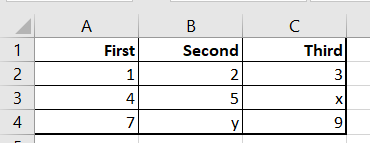Introduction

Read and Write numbers using MatlabShort introduction to managing numerical results using a txt file or a spreadsheet.

Live Script

Circle Length ApproximationExample of how to approximate the length of a circle by the perimeter of the inscribed polygon.

Live Script